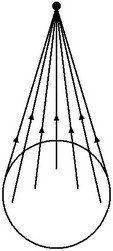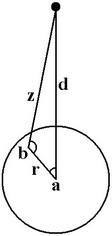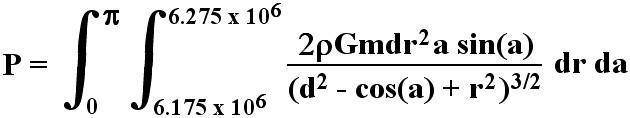# Astrophysics project gone wrong: why isn't the moon tearing the Earth apart?

• dBrandon/dC
In summary, the gravity of a large meteoroid passing by the Earth should be tearing the Earth apart. However, the pressure head of a column under gravity is the product of the medium's density, the gravitational acceleration, and the height of the column, and is affected by the distance from the gravitating object to every point within the asthenosphere. Additionally, the gravitational constant and the SIN of the angle are necessary to calculate the g factor.f

#### dBrandon/dC

I've been looking into meteorite impacts and large meteoroids passing by the Earth, and I started thinking about the physics of all that. And in the course of my playing around with the numbers, I found something that can't possibly be right, but I'm not sure what I'm doing wrong. Put simply, it looks like the gravity of the moon should be tearing the Earth apart.

... I started by considering the gravity of a large body passing near the Earth. Its gravity is going to pull on the planet (this is usually insignificant), but I realized it would also have effects in the Earth's mantle. Just for reference, the asthenosphere can be modeled as a sphere of viscous liquid sandwiched between two spheres of solid rock - the lithosphere above it and the solid mantle below it. It's around 100 km thick, and it begins about 200 km below the surface.

Anyway, I realized that the gravitational pull of a large, nearby object would have the following effect: its gravity would pull the asthenosphere directly below it straight up, but it would pull the asthenosphere of the rest of the planet at an angle. I'm including a picture to demonstrate this better.The gravitational force acting at angles to the Earth's radius would have two vector components - one pulling parallel to the radius, and one pulling perpendicular to it. This perpendicular component should act to pressurize the asthenosphere, pulling it everywhere around the planet in the direction of a point directly beneath the gravitating object. And with all of this pressure converging on one point (or rather, one infinitely thin line stretching from the top to the bottom of the asthenosphere), well, I think an appropriate analogy would be squeezing a tube of toothpaste. I think the pressure should try to force the viscous fluid either upward (and potentially through the crust) or downward (which I think is less likely, as the lower mantle is much more pressurized).

So I wanted to know exactly how much pressure we're talking about. Might it be enough, given a large enough object, to overcome the strength of the lithosphere and actually erupt volcanically? From a geophysical perspective, it's an interesting idea. But from a mathematical perspective, it's a little frightening. (I've actually posted my final equation on the calculus board here, hoping someone in that realm can help. But I'd like to know if you guys can find anything wrong with my physics, as well.)

I started with this. The pressure head of a column under gravity (P) is the product of the medium's density (ρ), the gravitational acceleration (g), and the height of the column (h). Thus,

Eq. 1: P = ρgh

The density of the asthenosphere is nearly constant at 3365 kg/m^3.
g will depend on the distance from the object to every point within the asthenosphere, as well as the angle of the gravitational force. The standard formula for g is:

Eq. 2: g = Gm/d^2

where G is the gravitational constant (6.67 x 10^-11 N m^2/kg^2),
m is the mass of the gravitating object (to be determined later),
and d is the distance from the object (also to be determined later).

I also needed to multiply this by the SIN of the angle to factor in the vector component of the angle. I defined the vertical in the picture as 0 radians. Thus, SIN(0) = 0, and directly under the object, its gravity would have no horizontal component (relative to the Earth's crust).

I decided to start by considering the asthenosphere as a 2-dimensional circle, and by considering one specific point therein, defined by r (the distance from the Earth's center) and a (the angle from the vertical).Referring to the second picture now, the distance from the gravitating object to the point in question (d in Equation 2 above; henceforth z, per the picture), can be found by:

Eq. 3: z = [d^2 + r^2 -2drCOS(a)]^1/2

The SIN of b can be found by:

Eq. 4: SIN(b) = dSIN(a)/z

Thus, the g factor of Equation 1 found by combining Equations 2, 3, and 4:

Eq. 5: g = GmdSIN(a)/z^3 = GmdSIN(a)/[d^2 + r^2 -2drCOS(a)]^3/2

For the height factor in Equation 1, again, I started by considering a 2-dimensional circle, whose circumference is given by:

Eq. 6: C = 2Пr

The "height" of the column, in this case, is actually going to be an arc with the fraction of the circumference given by:

Eq. 7: h = C(a/2П) = 2Пra/2П = ra

So now I have my density, acceleration due to gravity, and height for Equation 1. Putting together Equations 1, 5, and 7, we have:

Eq. 8: P = ρ * (GmdSIN(a)/[d^2 + r^2 -2drCOS(a)]^3/2) * (ra) = ρGmdraSIN(a)/[d^2 + r^2 -2drCOS(a)]^3/2

Now, so far, I've only been considering a 2-dimensional circle, where the asthenosphere is better modeled as a spherical area about 100 km thick. I decided to account for this in three ways. First, the area of the surface of a sphere is given by:

Eq. 9: A = 4Пr2

So to expand a circumference into the surface of a sphere, Equation 6 must be multiplied by 2r. Since there are trigonometry functions involved, I thought it would be best to only consider half the planet in the equation (a = 0 to П), and then simply double the result at the very end. So at this point, to expand the 2-dimensional circle into the surface of a sphere, I thought I only needed to account for an additional r. But as it turns out, I don't need to wait to double it. I still think it may be easier to only consider a from 0 to П, but it looks like the integration may not be affected either way. (I'm not sure if I'm correct in this.) So from this first consideration, Equation 8 becomes:

Eq: 10: P = 2ρGmdr^2 aSIN(a)/[d^2 + r^2 -2drCOS(a)]^3/2

Second, to account for the entire spherical surface, I need to integrate the equation for a = 0 to П. And third, to account for the thickness of the asthenosphere, I need to integrate it for r = 6.175 x 10^6 m to 6.275 x 10^6.

My final equation is in the picture here.Complicated, I know, but that's where I'm at. I don't think there's anything wrong with my physics, but there may be. I'm also unsure how to solve the equation. So I actually decided to make some simplifying assumptions and solve the equation, but the answer I got seemed way too high. That led me to considered the moon itself, just for the sake of comparison, and I still think the answer isn't anywhere close to right.

For the moon,

ρ = 3346 kg/m^3
m = 7.347 x 10^22 kg
d = 3.844 x 10^8 m

I decided to plug in r = 6.175 x 10^6 m and a = 30 deg (П/6 rad), and I arrived at the following:

From Equation 3, z = [d^2 + r^2 -2drCOS(a)]^1/2 = [1.434 x 10^17]^1/2 = 3.787 x 10^8 m

From Equation 4, SIN(b) = dSIN(a)/z = 0.5075; b = 149.5 deg (2.609 rad)

From Equation 5, g = GmdSIN(a)/[d^2 + r^2 -2drCOS(a)]^3/2 = 9.419 x 1020 / [1.434 x 10^17]^3/2 = 1.735 x 10^-5 m/s^2

And from Equation 7, h = ra = 3.233 x 10^6 m

Putting all of this into Equation 8, we have:

P = ρGmdraSIN(a)/[d^2 + r^2 -2drCOS(a)]^3/2 = 1.019 x 10^31 / [1.434 x 10^17]^3/2 = 1.877 x 10^5 Pascals

In other words, the pressure exerted on the central point directly under the moon from this column (where r = 6.175 x 10^6 m and the column height from a = П/6 rad to a = 0) is in the order of 188,000 Pascals of pressure.

I took this a bit farther and considered the moon's gravitational acceleration calculated above (g = 1.735 x 10-5 m/s^2) to be a decent enough average over the entire Earth, and I completely ignored the thickness of the asthenosphere (again, for the sake of simplicity). I then considered the height of the "column" (ra) for a = П , and I multiplied it all by 2r (that is, attempting to take in the entire sphere of the asthenosphere, albeit as an infinitely thin shell). I obtained:

P = 2ρr^2 a(1.735 x 10^-5 m/s^2) = 8.016 x 10^17 (1.735 x 10^-5 m/s^2) = 1.39 x 10^13 Pascals

In other words, as just a ballpark figure, the pressure caused by the moon in the asthenosphere seems to be somewhere around 14 trillion Pascals! By comparison, the pressure of the rocks of the lithosphere weighing down on the asthenosphere at that point, due to the Earth's gravity, is:

P = ρgh = (2700 kg/m^3)(9.8 m/s^2)(2 x 10^5 m) = 5.292 x 10^9 Pa

So the pressure caused by the moon is about 3,000 times the pressure caused by the weight of the rocks? Why doesn't the asthenosphere explode out of the Earth? Again, something is clearly wrong here. It may be my physics, but it may also be my math. And again, any assistance anyone can provide on this would be appreciated.

It is important to take the effect the moon has on the entire planet. The moon will exert a gravitational pull on the whole planet, so any net force will come from differences in this force. Pressure is just force per area, so any extra pressure will also arise from this difference in force.

Your equation for pressure is a statement of hydrostatic equilibrium (see wiki article). All quantities are related to the earth, including density enclosed in radius "h" and the gravitational acceleration. It is possible to proceed from here, but the effect of the moon is to reduce the effective gravitational acceleration, "g". At any point on earth, the total gravitational acceleration will be the acceleration from the Earth plus the acceleration from the moon. These two accelerations will add like vectors, as you indicated. You've already much of the legwork in this regard.

Fun facts, the moon raises a tide of about a meter in the Earth's ocean water. This is the ocean tidal height, since water is much more pliable than rock. The moon does pull the crust, but it is a very small amount (~cm maybe? I'm not sure).

Why doesn't the asthenosphere explode out of the Earth? Again, something is clearly wrong here. It may be my physics, but it may also be my math.

Brandon: The fact that the Earth is not being torn apart by the Moon is indeed a sign that you did something wrong.

It is important to take the effect the moon has on the entire planet. The moon will exert a gravitational pull on the whole planet, so any net force will come from differences in this force.
This is the key concept that Brandon missed.

Fun facts, the moon raises a tide of about a meter in the Earth's ocean water. This is the ocean tidal height, since water is much more pliable than rock. The moon does pull the crust, but it is a very small amount (~cm maybe? I'm not sure).
Sub-meter level, about 15 cm horizontally, 30 cm vertically.

Brandon: Do your calculations right and you should get something like the Earth tides. You will need to introduce some magic numbers that model where the Earth lies compared to the extremes of a perfectly rigid and perfectly fluid body. One set of such numbers are the Love numbers, named after A.E.H. Love. Unfortunately, googling "Love number" will mostly result in migarbages, most of which you do not want to open. You will have slightly better success googling the term "k2 Love number".

Here is a reference that goes into quite a bit of detail on the Earth tides:
http://www.gps.caltech.edu/classes/ge167/file/agnew_treat_tide.pdf

Last edited by a moderator:
My math is not good and I will not pretend to follow your calculations but did any of it take into account Earth's gravity pulling back on the asthenosphere?

Cheers

David